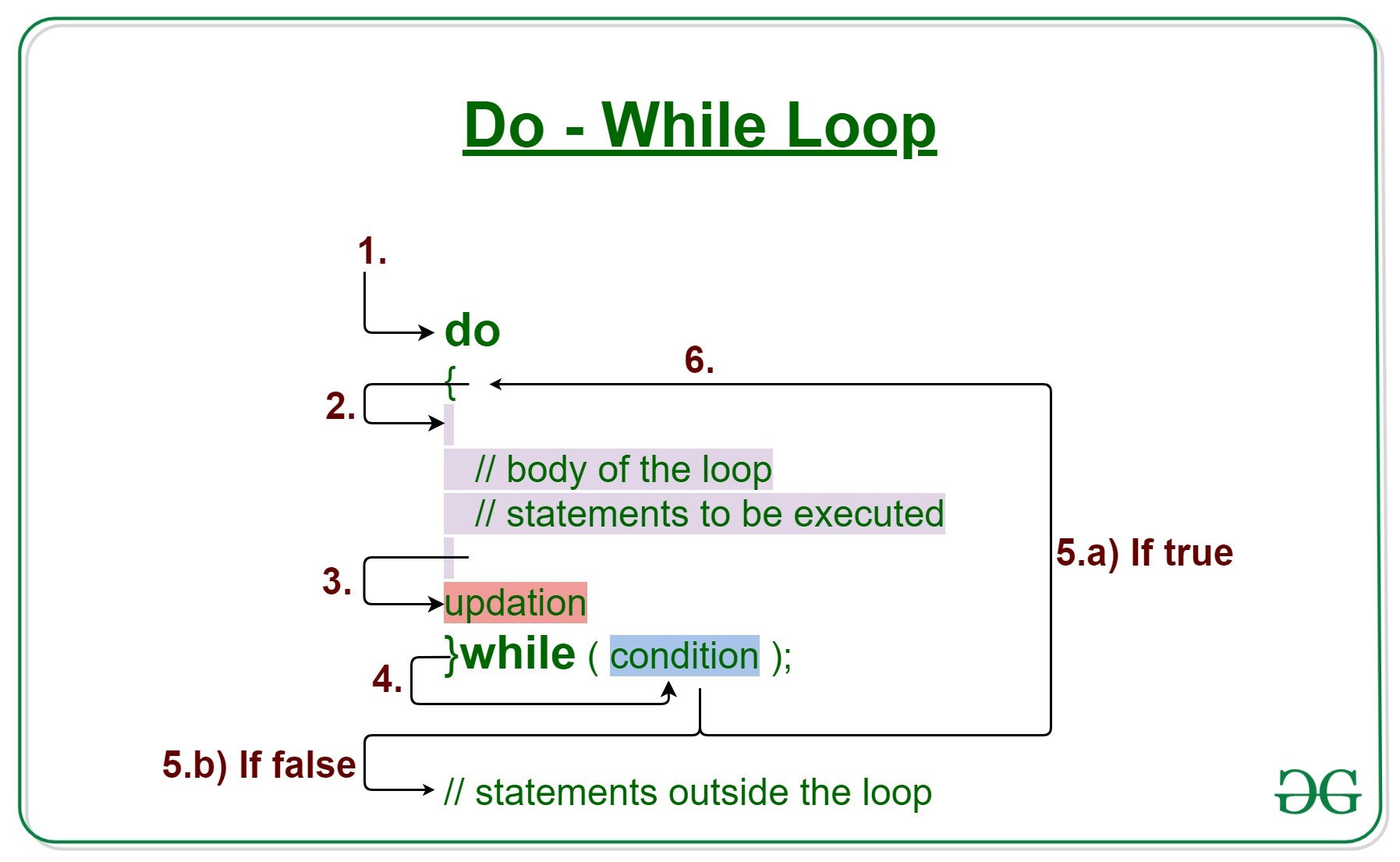# Java Program to Reverse a Number and find the Sum of its Digits Using do-while Loop

• Last Updated : 09 Mar, 2022

Problem Statement: The number is supposed to be entered by the user be it any random number lying within the primitive data-type holding the number. First, the number needs to be reversed. Secondary the sum of the number is to be calculated with the constraint to use a do-while loop.

do-while loop: Now the user sometimes does get confused between a while and a do-while loop. While loop checks the condition first and then executes the statement whereas the Do-while loop executes the statement then check the condition. An important point arising out from Do-while is even if the condition fails out still it will be executed once.Syntax of do-While Loop:

```do
{
// statement to be executed
}
while(condition check)```

Use of Do-while Loop:

Showing some menu to the user in other words the game is implemented where the goal is to show the user press 1 to do this, 2 to do that, and so on where there is an option press Q to quit this game. So do-while loop wants to show the menu at least once to the user. When the user has taken the action appropriate steps are done. So, while the condition should be if the user presses Q to quit and the menu is there inside the do-while loop.

Approach: Using do-while Loop, find the reverse of a number as well as the sum of its digits. Enter any number as an input and after that use modulus and division, operators to reverse that particular number and find the sum of its digits.

Algorithm:

1. Taking a number from the user
2. Creating two variables namely reverse_number and sum and initializing both of  them to 0
3. Reversing a number
4. Printing Reversed number
5. Printing the final sum of the digits as procured from smaller sums.

Reversal Algorithm as discussed as follows:

• Multiply rev by 10 and add remainder of number that is remainder to reverseNumber.  // rem = num%10 ; rev = rev*10 + rem;
• Add rem to the current sum to find the sum of digits and storing them in a variable that will be returned holding the final sum. Final_sum=current_sum where current_sum=current_sum + remainder
• Dividing number by 10 to access digit preceding the current digit of the number as follows. // num=num/10; while(num > 0);

Example 1: Java program to reverse and sum up digits of the number without making functions

## Java

 `// Java program to reverse and and sum up digits of number` `// Importing generic Classes/Files``import` `java.io.*;``// Importing Scanner Class``import` `java.util.Scanner;` `class` `GFG {` `    ``// Main driver method``    ``public` `static` `void` `main(String[] args)``    ``{``        ``// creating variables``        ``int` `num, rem;` `        ``// Creating variables and initializing at same time``        ``int` `rev = ``0``, sum = ``0``;` `        ``// Using scanner to take input from user``        ``// Scanner sc = new Scanner(System.in);` `        ``// Remove comments from lineNo20``        ``// to take dynamic user input` `        ``// Displaying message``        ``System.out.println(``"Enter the number: 25 "``);` `        ``// Taking input from user``        ``// num = sc.nextInt();` `        ``// Hard coded input``        ``num = ``25``;` `        ``// Do-while loop for iteration over digits of number``        ``do` `{` `            ``// Step1: Modulo with 10 to get last digit``            ``rem = num % ``10``;` `            ``// Step2: Reverse the number``            ``rev = rev * ``10` `+ rem;` `            ``// Sum of the digits of number``            ``sum = sum + rem;` `            ``// Step3: Dividing number by 10 to loose last``            ``// digit``            ``num = num / ``10``;``        ``}` `        ``// Condition check``        ``// Remember: By this time 1 iteration is over even``        ``// if conditions false``        ``while` `(num > ``0``);` `        ``// Printing the reverse number``        ``System.out.println(``"Reverse of given number: "``                           ``+ rev);` `        ``// Summing up digits of number as shown in above``        ``// steps``        ``System.out.println(``"Sum of digits of given number: "``                           ``+ sum);``    ``}``}`

Output

```Enter the number: 25
Reverse of given number: 52
Sum of digits of given number: 7```

Example 2: In this example separately do-while loops are shown as an illustration by creating functions of them and later on calling them in the main driver method.

## Java

 `import` `java.io.*;` `// Importing specific Scanner Class to show menu``import` `java.util.Scanner;` `class` `GFG {` `    ``// Iterative function to reverse digits of number``    ``// static int reversDigits(int num)` `    ``// Hardcoded input where input number equals 25``    ``static` `int` `reversDigits(``int` `num)``    ``{` `        ``// Making input hard coded else``        ``num = ``25``;``        ``// else do not initialize num` `        ``// creating and Initialising reverseNo with 0``        ``// Creating remainder variable``        ``int` `rev = ``0``, rem;` `        ``// Statements to be executed in do loop``        ``do` `{``            ``// Reversal of a number as discussed in``            ``// algorithm``            ``rem = num % ``10``;``            ``rev = rev * ``10` `+ rem;``            ``num = num / ``10``;` `        ``}` `        ``// Condition check``        ``while` `(num > ``0``);` `        ``// Returning reverse of the user enter number``        ``return` `rev;``    ``}` `    ``// Iterative function findingsum of the digits of number``    ``// static int sumDigits(int num)` `    ``// Hardcoded input where input number equals 25``    ``static` `int` `sumDigits(``int` `num)``    ``{``        ``// Making input hard coded``        ``num = ``25``;``        ``// else do not initialize num` `        ``// creating and Initialising final_sum with 0``        ``// Creating remainder variable``        ``int` `sum = ``0``, rem;` `        ``// Statements to be executed in do loop``        ``do` `{``            ``// Retrieving steps as discussed in above 3``            ``// steps``            ``rem = num % ``10``;``            ``sum = sum + rem;``            ``num = num / ``10``;` `        ``}``        ``// condition check``        ``while` `(num > ``0``);` `        ``// Returning Sum of digits of a reversed number``        ``return` `sum;``    ``}` `    ``// Main driver method``    ``public` `static` `void` `main(String[] args)``    ``{``        ``// declaring variable to store user entered number``        ``// int num;` `        ``// Scanner Class to read the entered number` `        ``// Commented out to hard code the input``        ``// else remove comments``        ``// Scanner sc = new Scanner(System.in);` `        ``// Input is hardcoded for display``        ``// else remove comments from line no 77` `        ``// Considering hard coded input``        ``int` `num = ``25``;` `        ``// Printing message``        ``System.out.println(num);` `        ``// Taking input from user``        ``// Making input hard coded else``        ``// remove comments from line no 82``        ``// num = sc.nextInt();` `        ``// Calling above functions to print reversed number``        ``System.out.println(``"Reverse of given number: "``                           ``+ reversDigits(num));` `        ``// Calling above functions to print reversed number``        ``System.out.println(``"Sum of digits of given number: "``                           ``+ sumDigits(num));``    ``}``}`

Output

```25
Reverse of given number: 52
Sum of digits of given number: 7```

My Personal Notes arrow_drop_up